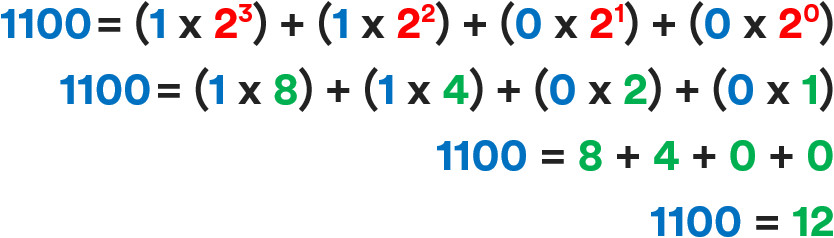﻿ Binary Numbers: An Introduction
Note #4
Binary Numbers: An Introduction

Binary - a number system used to encode information using 0s and 1s - lies at the heart of every digital computer.

What is binary?

Binary is a base-2 number system, which only uses two digits (0 & 1). It is a system used at the heart of every digital computer, allowing them to encode information, perform arithmetic operations and execute logical control processes.

Using two digits as opposed to, say, the familiar ten digits used in decimal systems (0 to 9) enables hardware to be easily implemented via simple ‘on’ or ‘off’ circuit states or logic gates. This is the basis for all digital systems.

Understanding binary numbers

To understand binary values imagine each digit (or ‘bit’) of the binary notation as representing an increasing power of 2 - with the rightmost digit representing 20, the next representing 21, then 22 and so on.

For each bit, the 1 or 0 signifies whether the value of the increasing power of two summates towards the number's total.

As a visual example, figure 2 shows the binary value 1100 converted into the decimal value 12. Blue numbers represent the binary notation, red numbers represent the increasing powers of 2, and green numbers represent the decimal values.Figure 2. Binary to decimal: representation of 12.

Binary values are often represented in different ‘bit’ lengths or ‘word’ sizes. In the example above the value is represented in 4-bits, called a ‘nibble’. This means a value between 0 and 15 can be represented. 8-bit values, referred to as ‘bytes’ can represent a value between 0 and 255. Figure 3 shows the 4-bit binary representation for each decimal value between 0 and 15.

Learn more:

To learn more about computational hardware, why not checkout our next note: Binary Addition with Full Adders.

Explore our DIY Electronic Kits: